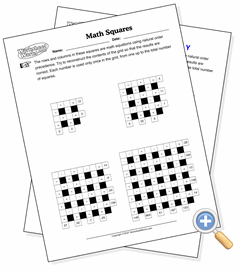# Math SquaresDevelop problem solving strategies with math

A math square is a set of equations that have been laid out in rows and columns so that the numbers in each equation intersect. Between each row and column are the operations (addition, subtraction, multiplication and division) which define the equations in the columns and rows.

There are three types of ways you can set up the math square. The first is to populate it with all of the values and operations, and let the student compute the result. This is the easiest and most straightforward task. A real math square puzzle, however, is where the results and operations are given, and the student must derive the values that plug into each equation to get the result. This is much more challenging, and is nearly impossible with the 5x5 puzzle (at this point making it a computer programming exercise might be more relevant). A third option is to have the values and results present, but let the student figure out what the operation is. This is somewhat easier since there are only four possible results, and division operations can be spotted easily since the result of any division operation needs to be an integer.